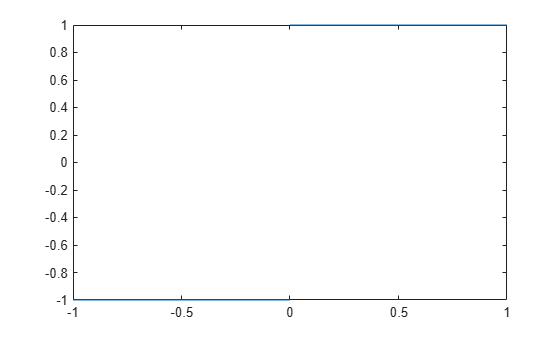Documentation

## Limits

The fundamental idea in calculus is to make calculations on functions as a variable “gets close to” or approaches a certain value. Recall that the definition of the derivative is given by a limit

`$f\text{'}\left(x\right)=\underset{h\to 0}{\mathrm{lim}}\frac{f\left(x+h\right)-f\left(x\right)}{h},$`

provided this limit exists. Symbolic Math Toolbox™ software enables you to calculate the limits of functions directly. The commands

```syms h n x limit((cos(x+h) - cos(x))/h, h, 0)```

which return

```ans = -sin(x)```

and

`limit((1 + x/n)^n, n, inf)`

which returns

```ans = exp(x)```

illustrate two of the most important limits in mathematics: the derivative (in this case of cos(x)) and the exponential function.

### One-Sided Limits

You can also calculate one-sided limits with Symbolic Math Toolbox software. For example, you can calculate the limit of x/|x|, whose graph is shown in the following figure, as x approaches 0 from the left or from the right.

```syms x fplot(x/abs(x), [-1 1], 'ShowPoles', 'off')```To calculate the limit as x approaches 0 from the left,

`$\underset{x\to {0}^{-}}{\mathrm{lim}}\frac{x}{|x|},$`

enter

```syms x limit(x/abs(x), x, 0, 'left')```
```ans = -1```

To calculate the limit as x approaches 0 from the right,

`$\underset{x\to {0}^{+}}{\mathrm{lim}}\frac{x}{|x|}=1,$`

enter

```syms x limit(x/abs(x), x, 0, 'right')```
```ans = 1```

Since the limit from the left does not equal the limit from the right, the two- sided limit does not exist. In the case of undefined limits, MATLAB® returns `NaN` (not a number). For example,

```syms x limit(x/abs(x), x, 0)```

returns

```ans = NaN```

Observe that the default case, `limit(f)` is the same as `limit(f,x,0)`. Explore the options for the `limit` command in this table, where `f` is a function of the symbolic object `x`.

Mathematical Operation

MATLAB Command

$\underset{x\to 0}{\mathrm{lim}}f\left(x\right)$

`limit(f)`

$\underset{x\to a}{\mathrm{lim}}f\left(x\right)$

`limit(f, x, a) or`

`limit(f, a)`

$\underset{x\to a-}{\mathrm{lim}}f\left(x\right)$

`limit(f, x, a, 'left')`

$\underset{x\to a+}{\mathrm{lim}}f\left(x\right)$

`limit(f, x, a, 'right')`

#### Mathematical Modeling with Symbolic Math Toolbox

Get examples and videos